# Integer Value of Ray and Wave Optics, Past year Questions JEE Advance, Class 12, Physics JEE Notes | EduRev

## JEE : Integer Value of Ray and Wave Optics, Past year Questions JEE Advance, Class 12, Physics JEE Notes | EduRev

The document Integer Value of Ray and Wave Optics, Past year Questions JEE Advance, Class 12, Physics JEE Notes | EduRev is a part of the JEE Course Class 12 Physics 35 Years JEE Mains &Advance Past year Paper.
All you need of JEE at this link: JEE

Q.1. The focal length of a thin biconvex lens is 20 cm. When an object is moved from a distance of 25 cm in front of it to 50 cm, the magnification of its image changes from m25 to m50. The ratio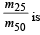(2010)

Ans. 6

Solution.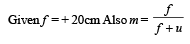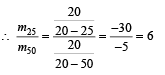Q.2. A large glass slab (μ= 5 / 3) of thickness 8 cm is placed over a point source of light on a plane surface. It is seen that light emerges out of the top surface of the slab from a circular area of radius R cm. What is the value of R?     (2010)

Ans. 6

Solution. In the figure, C represents the critical angle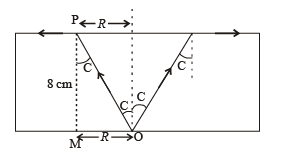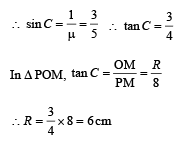Q.3. Image of an object approaching a convex mirror of radius of curvature 20 m along its optical axis is observed to move from 25/3 m to 50/7 m in 30 seconds. What is the speed of the object in km per hour?  (2010)

Ans. 3

Solution. Using mirror formula for first position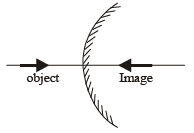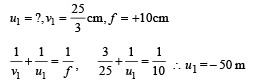Using mirror formula for the second position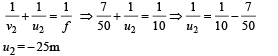Change in position of object = 25 m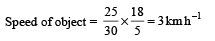Q.4. Water (with refractive index = 4/3) in a tank is 18 cm deep. Oil of refractive index 7/4 lies on water making a convex surface of radius of curvature ‘R = 6 cm’ as shown. Consider oil to act as a thin lens. An object ‘S’ is placed 24 cm above water surface. The location of its image is at ‘x’ cm above the bottom of the tank. Then ‘x’ is     (2011)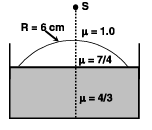Ans. 2

Solution. For the convex spherical refracting surface of oil we apply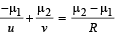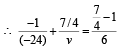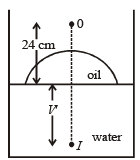∴ v = 21 cm

For water-oil interface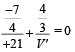∴ V' = 16 cm.

This is the image distance from water-oil interface.

Therefore the distance  of the image from the bottom of the tank is 2 cm.

Q.5. A Young's double slit interference arrangement with slits S1 and S2 is immersed in water (refractive index = 4/3) as shown in the figure. The positions of maximum on the surface of water are given by x2 = p2m2λ2 – d2, where l is the wavelength of light in air (refractive index = 1), 2d is the separation between the slits and m is an integer. The value of p is      (JEE Adv. 2015)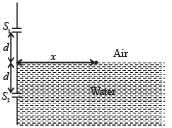Ans. 3

Solution. For maxima

Path defference = mλ

∴ S2A – S1A = mλ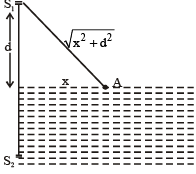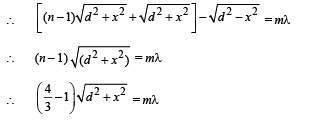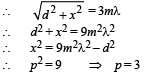Q.6. Consider a concave mirror and a convex lens (refractive index = 1.5) of focal length 10 cm each, separated by a distance of 50 cm in air (refractive index = 1) as shown in the figure. An object is placed at a distance of 15 cm from the mirror. Its erect image formed by this combination has magnification M1. When the set-up is kept in a medium of refractive index 7/6, the magnification becomes M2. The magnitude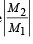is         (JEE Adv. 2015)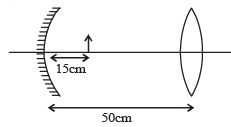Ans. 7

Solution. Applying mirror formula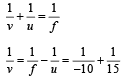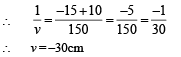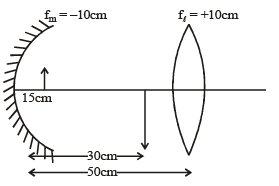For convex lens u = |2f

Therefore image will have a magnification of 1.

When the set – up is kept in a medium

The focal length of the lens will change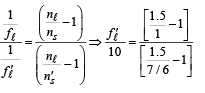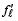= 17.5 cm.

Applying lens formula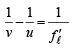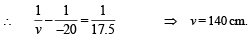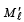= Magnification by lens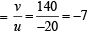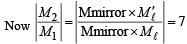Q.7. The monochromatic beam of light is incident at 60° on one face of an equilateral prism of refractive index n and emerges from the opposite face making an angle θ(n) with the normal (see the figure). For n = √3 the value of q is 60° and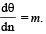The value of m is                     (JEE Adv. 2015)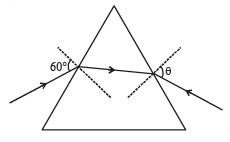Ans. 2

Solution. Here ∠MPQ + ∠MQP  = 60°. If ∠MPQ = r then ∠MQP

= 60 – r

Applying Snell’s law at P

sin60° = n sin r                    ...(i)

Differentiating w.r.t ‘n’ we get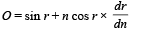...(ii)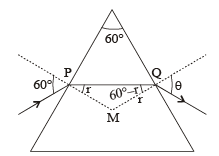Applying Snell’s law at Q

sin θ = n sin (60° – r) ...(iii)

Differentiating the above equation w.r.t ‘n’ we get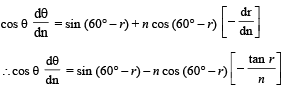[from (ii)]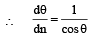[sin (60° – r) + cos (60° – r) tan r] ...(iv)

From eq. (i), substituting n = √3 we get r = 30°

From eq (iii), substituting n = √3 , r = 30° we get θ = 60°

On substituting the values of r and θ in eq (iv) we get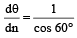[sin 30° + cos 30° tan 30°] = 2

Offer running on EduRev: Apply code STAYHOME200 to get INR 200 off on our premium plan EduRev Infinity!

42 docs|19 tests

,

,

,

,

,

,

,

,

,

,

,

,

,

,

,

,

,

,

,

,

,

,

,

,

,

,

,

,

,

,

;